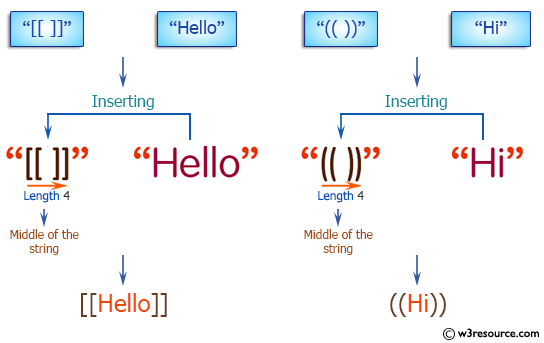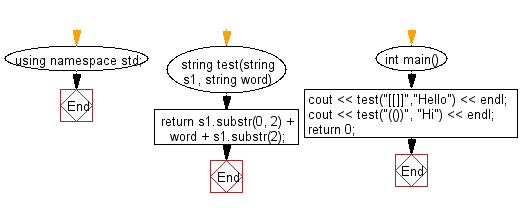﻿ C++ : Insert a string into the middle of another string# C++ Exercises: Insert a given string into middle of the another given string of length 4

## C++ Basic Algorithm: Exercise-58 with Solution

Write a C++ program to insert a given string into middle of the another given string of length 4.

Sample Solution:

C++ Code :

``````#include <iostream>

using namespace std;

string test(string s1, string word)
{
return s1.substr(0, 2) + word + s1.substr(2);
}

int main()
{
cout << test("[[]]","Hello") << endl;
cout << test("(())", "Hi") << endl;
return 0;
}
``````

Sample Output:

```[[Hello]]
((Hi))
```

Pictorial Presentation:Flowchart:C++ Code Editor: